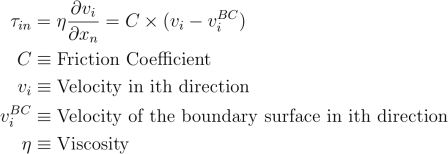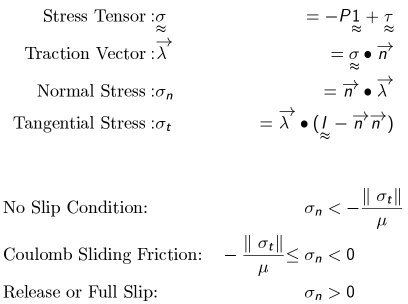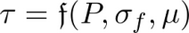﻿ Friction Models
Manufacturing Solutions

# Friction Models# Friction ModelsThe HX solver supports five different friction models. They are:

 • Stick
 • Slip
 • SlipVelocity
 • Coulomb
 • Viscoplastic

## Stick

This is also referred to as “full stick” and it implies that the moving material particle adheres to the boundary surface and takes the value of the velocity of the wall.  That is, if the boundary surface is stationary (Vx=0, Vy=0, Vz=0) – then the material particle also assumes the same velocity. This means the Dirichlet boundary condition (also known as essential boundary condition) of (Vx=0, Vy=0, Vz=0) is applied at these boundary surfaces for the momentum equations.  This BC is often used with Newtonian and non-Newtonian fluids.  We use the stick BC in container region for metal extrusion.

## Slip

Material particles completely slip over the boundary surface, hence, this is also denoted as “full slip.”  Velocity of the boundary surface has no effect on the deforming material. We rarely use this BC. When this BC is applied, the Neumann condition that the three tractions are zero together with the condition that material cannot flow the boundary surface (normal velocity = 0) is applied.

## Slip Velocity

This model specifies the frictional shear stress at the wall as a linear function of the slip velocity. Value of the friction coefficient is dependent on the material viscosity and the length scale. A value of 1.0E+04 may be adequate for water to stick to the walls, however, the same value will cause a very viscous material to slip completely. If you use the model for metal extrusion (aluminum), the value specified should be greater than 1.0E+09 – anything below this will cause the material to slip.## Coulomb Friction

Coulomb friction is a dynamic friction model unlike the slip velocity model. This model can exhibit all three states of friction:

 • Stick – deforming material sticks to the boundary surface and assumes the boundary velocity.
 • Sliding Friction – deforming material slides on the boundary according to Coulomb friction model.
 • Release – deforming material slips on the boundary and does not suffer any tangential velocity gradient.  This is same as full slip.

This model can exhibit slip - stick instability or resolve itself to full slip or stick. In addition, the dynamic nature of the friction condition can slow down convergence.## ViscoPlastic

This model is similar to Coulomb and the main difference is the shear stress is a function of pressure and flow stress. Value of the friction coefficient is similar to the Coulomb model (0 = slip, 1= full stick, default is 0.30).Unlike the Coulomb model, this is not prone to oscillation. However, it is can also tend to full stick or full slip for some cases. HX uses a proprietary version of this model and it is customized to accurately capture the friction behavior on boundary surfaces with bearing profile.